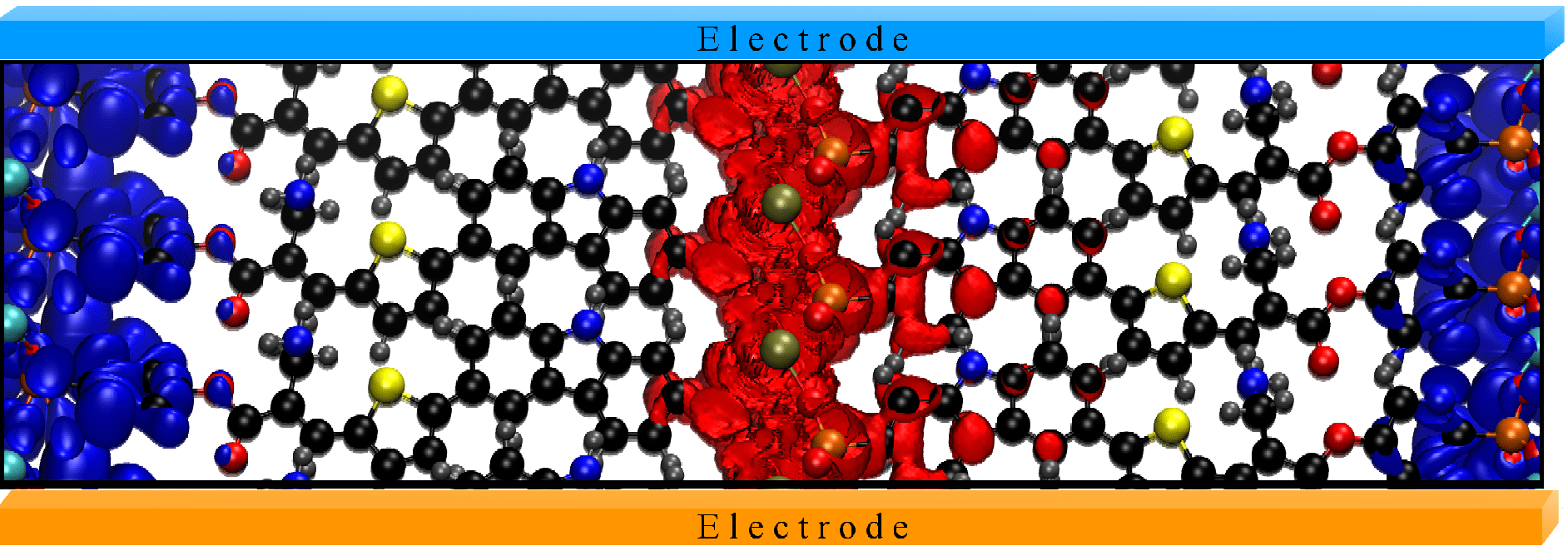# Cube File Generation for Energy Range

Good morning all,

Something that Quantum Espresso [pwscf] really bugs me is that it is annoyingly difficult program to generate a plot of the CBM or the VBM of a structure. This is something that programs like NwChem or GUASSIAN can do with a little switch. I created a little Python script that can generate all the input files necessary to generate the final cube file.

First, some background: pwscf comes with a nifty little post processing program called pp.x. The documentation for it, like almost everything involving computational physics, is pretty poor. Here is the basic input file that we will be using:

```
&inputpp
prefix='../MK21xThio'
outdir = '.'
filplot='22.282.plot'
plot_num = 7
kpoint=22
kband=282
/
&plot
/

```

You can find the whole definition here: http://www.quantum-espresso.org/wp-content/uploads/Doc/INPUT_PP.html. If you want to plot the data for an energy range, you will need to run plot_num = 7 with pp.x (this means it extracts the charge density associated with a given kpoint and kband). In this example file, 22.282.plot will contain all the information for the 22nd kpoint and 282nd band in this system. If you want to plot the energy range between Emin < E < Emax, you need to generate a *.plot file for each kpoint and each kband within the energy range. As you can imagine this can lead to a lot of tedious work.

To automate this task, I have created the following python script. You can download it here: http://levilentz.com/Codes/eig_extractor.py. This code runs within the *.save directory and will output a slew of files that need to be computed with pp.x. If you are on a time crunch, running only one of the .in files per kpoint will give you a pretty good idea of what is happening in that energy region. The code is relatively simple (just text parsing of xml files), so I will not labour to explain the minutia. If you have big issues with it, please contact me (email is in the source).

The way I recommend running it is in a folder specifically for each of these files:

`pp.x < Kpoint.Kband.in > Kpoint.Kband.out `

After this, you will have each of the *.plot files necessary to be combined. The code also creates a plotting file (to combine all the *.plot files into a single *.cube file). An example is given below:

```
&inputpp
/
&plot
nfile = 36
filepp(1) =1.281.plot
filepp(2) =2.281.plot
filepp(3) =3.281.plot
filepp(4) =4.281.plot
filepp(5) =5.281.plot
filepp(6) =6.281.plot
filepp(7) =7.281.plot
filepp(8) =8.281.plot
filepp(9) =9.281.plot
filepp(10) =10.281.plot
filepp(11) =11.281.plot
filepp(12) =12.281.plot
filepp(13) =13.281.plot
filepp(14) =14.281.plot
filepp(15) =15.281.plot
filepp(16) =16.281.plot
filepp(17) =17.281.plot
filepp(18) =18.281.plot
filepp(19) =19.281.plot
filepp(20) =20.281.plot
filepp(21) =21.281.plot
filepp(22) =22.281.plot
filepp(23) =23.281.plot
filepp(24) =24.281.plot
filepp(25) =25.281.plot
filepp(26) =26.281.plot
filepp(27) =27.281.plot
filepp(28) =28.281.plot
filepp(29) =29.281.plot
filepp(30) =30.281.plot
filepp(31) =31.281.plot
filepp(32) =32.281.plot
filepp(33) =33.281.plot
filepp(34) =34.281.plot
filepp(35) =35.281.plot
filepp(36) =36.281.plot
weight(1) = 0.0277777777778
weight(2) = 0.0277777777778
weight(3) = 0.0277777777778
weight(4) = 0.0277777777778
weight(5) = 0.0277777777778
weight(6) = 0.0277777777778
weight(7) = 0.0277777777778
weight(8) = 0.0277777777778
weight(9) = 0.0277777777778
weight(10) = 0.0277777777778
weight(11) = 0.0277777777778
weight(12) = 0.0277777777778
weight(13) = 0.0277777777778
weight(14) = 0.0277777777778
weight(15) = 0.0277777777778
weight(16) = 0.0277777777778
weight(17) = 0.0277777777778
weight(18) = 0.0277777777778
weight(19) = 0.0277777777778
weight(20) = 0.0277777777778
weight(21) = 0.0277777777778
weight(22) = 0.0277777777778
weight(23) = 0.0277777777778
weight(24) = 0.0277777777778
weight(25) = 0.0277777777778
weight(26) = 0.0277777777778
weight(27) = 0.0277777777778
weight(28) = 0.0277777777778
weight(29) = 0.0277777777778
weight(30) = 0.0277777777778
weight(31) = 0.0277777777778
weight(32) = 0.0277777777778
weight(33) = 0.0277777777778
weight(34) = 0.0277777777778
weight(35) = 0.0277777777778
weight(36) = 0.0277777777778

iflag = 3
output_format = 6
fileout = 'VB.cube'
/

```

This needs to be run after all the plot files are generated. The weights are calculated a 1/#kpoints, where this might not be 100% necessary and you can tweak this in the *.py file. If you run this with the normal pp.x file, you will get the following error:

`nfile is too large, exiting`

The only reason I can tell is that the pp.x program requires a lot of memory, so they wanted to limit the number of files that pp.x had to maintain in memory space. In order to remove this restriction, edit the file chdens.f90, changing

`INTEGER, PARAMETER :: nfilemax = 7`

to

`<INTEGER, PARAMETER :: nfilemax = 7`

or some number that is large, then recompile pp.x. Any modern server will be able to handle a large number of plot files. You should only need to worry about this if your system has a low amount of available memory. I have tested this with approximately 200 plot files on a system with 64GB of ram and it works flawlessly.

Once you generate that file, you can create cool plots like this:Ok, it is still a working image. Dont worry, my adviser will yell at me for it.